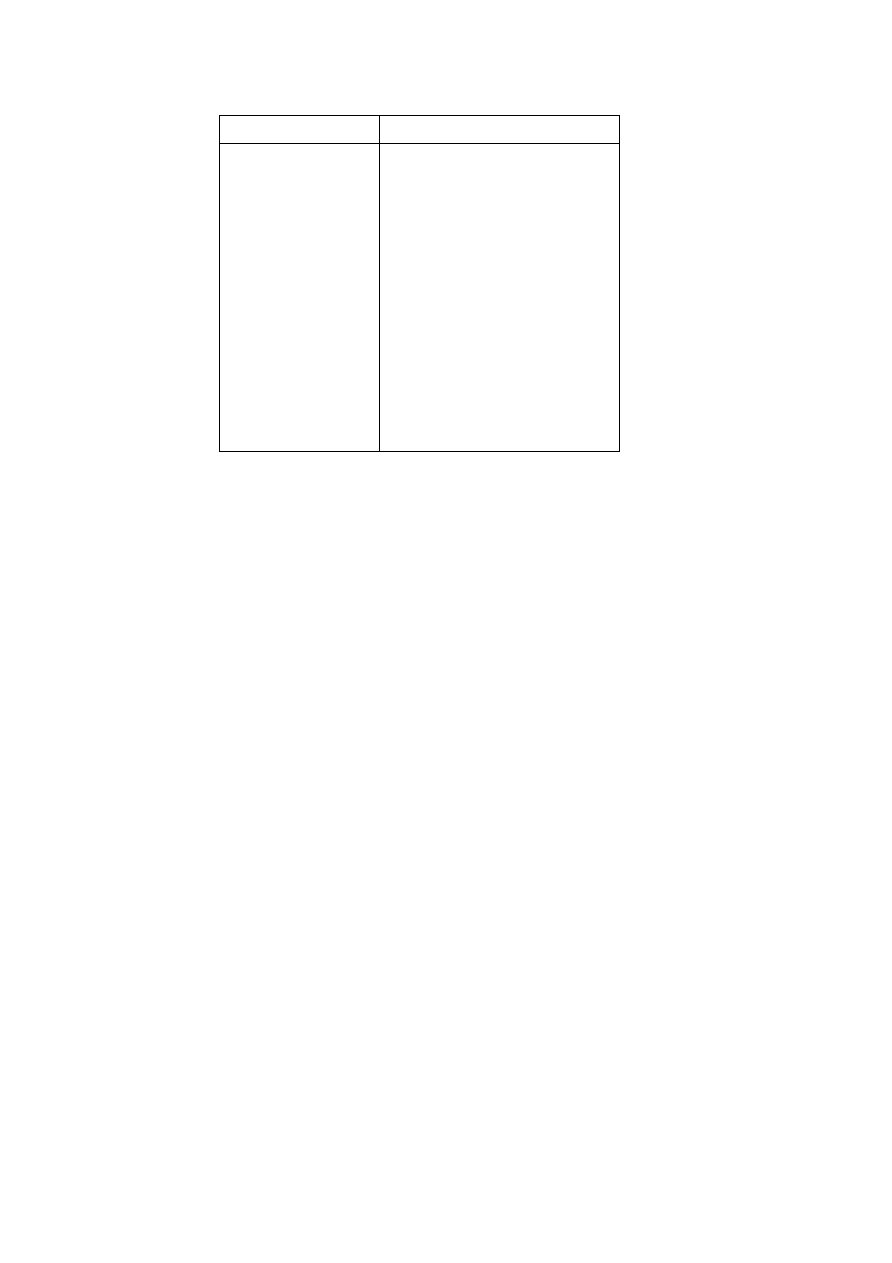ISO/IEC 10918-1 : 1993(E)
Table F.1 ­ Difference magnitude categories for DC coding
SSSS
DIFF values
1
0
0
1
1
­1,1
1
2
­3,­2,2,3
1
3
­7..­4,4..7
1
4
­15..­8,8..15
1
5
­31..­16,16..31
1
6
­63..­32,32..63
1
7
­127..­64,64..127
1
8
­255..­128,128..255
1
9
­511..­256,256..511
10
­1 023..­512,512..1 023
11
­2 047..­1 024,1 024..2 047
F.1.2.1.3
Huffman encoding procedures for DC coefficients
The encoding procedure is defined in terms of a set of extended tables, XHUFCO and XHUFSI, which contain the
complete set of Huffman codes and sizes for all possible difference values. For full 12-bit precision the tables are relatively
large. For the baseline system, however, the precision of the differences may be small enough to make this description
practical.
XHUFCO and XHUFSI are generated from the encoder tables EHUFCO and EHUFSI (see Annex C) by appending to the
Huffman codes for each difference category the additional bits that completely define the difference. By definition,
XHUFCO and XHUFSI have entries for each possible difference value. XHUFCO contains the concatenated bit pattern of
the Huffman code and the additional bits field; XHUFSI contains the total length in bits of this concatenated bit pattern.
Both are indexed by DIFF, the difference between the DC coefficient and the prediction.
The Huffman encoding procedure for the DC difference, DIFF, is:
SIZE
=
XHUFSI(DIFF)
CODE
=
XHUFCO(DIFF)
code SIZE bits of CODE
where DC is the quantized DC coefficient value and PRED is the predicted quantized DC value. The Huffman code
(CODE) (including any additional bits) is obtained from XHUFCO and SIZE (length of the code including additional
bits) is obtained from XHUFSI, using DIFF as the index to the two tables.
F.1.2.2
Huffman encoding of AC coefficients
F.1.2.2.1
Structure of AC code table
Each non-zero AC coefficient in ZZ is described by a composite 8-bit value, RS, of the form